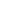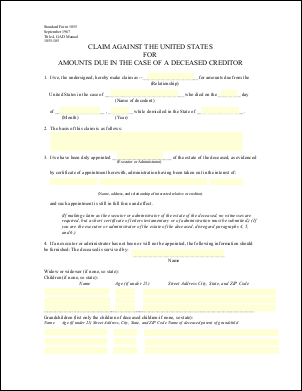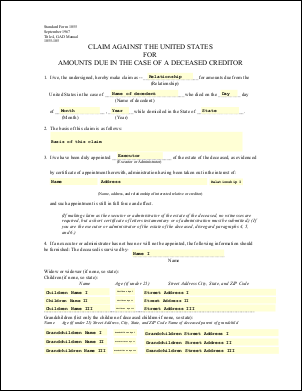eForm Fields ExampleThis example shows how to change the values of eForm fields. In this example we simply replace each of the fields in a form with the name of that field.Src

 First we create an ABCpdf Doc object and read in our template form.   ```using (Doc doc = new Doc()) {   doc.Read(Server.MapPath("../mypics/form.pdf"));   doc.Form.NeedAppearances = false; // for PDF 2.0``` ```Using doc As New Doc()   doc.Read(Server.MapPath("../mypics/form.pdf"))   doc.Form.NeedAppearances = False ' for PDF 2.0 ```We iterate through each of the top level fields. For each field we set the value of the field to be equal to the value of the name.   ```  string[] theNames = doc.Form.GetFieldNames();   foreach (string theName in theNames) {     Field theField = doc.Form[theName];     theField.Value = theField.Name;   } ``` ```  Dim theNames As String() = doc.Form.GetFieldNames()   For Each theName As String In theNames     Dim theField As Field = doc.Form(theName)     theField.Value = theField.Name   Next ```Finally we save.   ```  doc.Save(Server.MapPath("eformfields.pdf")); } ``` ```  doc.Save(Server.MapPath("eformfields.pdf")) End Using ```Given the following document.form.pdf This is the kind of output you might expect.eformfields.pdf